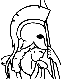#Constrained Optimization and Lagrange Multiplier Methods Table of Contents:

1. Introduction

1. General Remarks
2. Notation and Mathematical Background
3. Unconstrained Optimization
1. Convergence Analysis of Gradient Methods
2. Steepest Descent and Scaling
3. Newton's Method and Its Modifications
4. Conjugate Direction and Conjugate Gradient Methods
5. Quasi-Newton Methods
6. Methods not Requiring Evaluation of Derivatives
4. Constrained Minimization
5. Algorithms for Minimization Subject to Simple Constraints
6. Notes and Sources

2. The Method of Multipliers for Equality Constrained Problems

1. The Quadratic Penalty Function Method
2. The Original Method of Multipliers
1. Geometric Interpretation
2. Existence of Local Minima of the Augmented Lagrangian
3. The Primal Functional
4. Convergence Analysis
5. Comparison with the Penalty Method - Computational Aspects
3. Duality Framework for the Method of Multipliers
1. Stepsize Analysis for the Method of Multipliers
2. The Second-Order Multiplier Iteration
3. Quasi-Newton Versions of the Second-Order Iteration
4. Geometric Interpretation of the Second-Order Multiplier Iteration
4. Multiplier Methods with Partial Elimination of Constraints
5. Asymptotically Exact Minimization in the Method of Multipliers
6. Primal-Dual Methods Not Utilizing a Penalty Function
7. Notes and Sources

3. The Method of Multipliers for Inequality Constrained and Nondifferentiable Optimization Problems

1. One-Sided Inequality Constraints
2. Two-Sided Inequality Constraints
3. Approximation Procedures for Nondifferentiable and Ill-Conditioned Optimization Problems
4. Notes and Sources

4. Exact Penalty Methods and Lagrangian Methods

1. Nondifferentiable Exact Penalty Functions
2. Linearization Algorithms Based on Nondifferentiable Exact Penalty Functions
1. Algorithms for Minimax Problems
2. Algorithms for Constrained Optimization Problems
3. Differentiable Exact Penalty Functions
1. Exact Penalty Functions Depending on x and lambda
2. Exact Penalty Functions Depending Only on x
3. Algorithms Based on Differentiable Exact Penalty Functions
4. Lagrangian Methods - Local Convergence
1. First-Order Methods
2. Newton-like Methods for Equality Constraints
3. Newton-like Methods for Inequality Constraints
4. Quasi-Newton Versions
5. Lagrangian Methods - Global Convergence
1. Combinations with Penalty and Multiplier Methods
2. Combinations with Differentiable Exact Penalty Methods - Newton and Quasi-Newton Versions
3. Combinations with Nondifferentiable Exact Penalty Methods - Powell's Variable Metric Approach
6. Notes and Sources

5. Nonquadratic Penalty Functions - Convex Programming

1. Classes of Penalty Functions and Corresponding Methods of Multipliers
1. Penalty Functions for Equality Constraints
2. Penalty Functions for Inequality Constraints
3. Approximation Procedures Based on Nonquadratic Penalty Functions
2. Convex Programming and Duality
3. Convergence Analysis of Multiplier Methods
4. Rate of Convergence Analysis
5. Conditions for Penalty Methods to be Exact
6. Large Scale Integer Programming Problems and the Exponential Method of Multipliers
1. An Estimate of the Duality Gap
2. Solution of the Dual and Relaxed Problems
7. Notes and Sources

6. References

7. Index